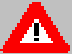### JPL's Wireless Communication Reference Website

#### Chapter: Cellular Telephone Networks Section: Outage probability# Outage Probability in Rayleigh-Fading ChannelsThis pages discusses a Pascal program that computes the capture and outage probability in cellular mobile radio channel with

## Definition of some constants

First of all we define the following constants:
```Var
HER          : array[1 .. 2, 1 .. 20] of real;
N            : Integer
rootpiinv, ln10: real

BEGIN
rootpiinv   :=  1/(sqrt(3.141592));
N           :=  20;
ln10        := ln(10);

HER[1, 1]   :=  0.2453407083;
HER[1, 2]   :=  0.7374737285;
HER[1, 3]   :=  1.2340762153;
HER[1, 4]   :=  1.7385377121;
HER[1, 5]   :=  2.25497400203;
HER[1, 6]   :=  2.78880605844;
HER[1, 7]   :=  3.34785456736;
HER[1, 8]   :=  3.94476404017;
HER[1, 9]   :=  4.603682449510;
HER[1,10]   :=  5.387480890013;

HER[2, 1]   :=  4.6224366960E-1;
HER[2, 2]   :=  2.8667550536E-1;
HER[2, 3]   :=  1.0901720602E-1;
HER[2, 4]   :=  2.4810520887E-2;
HER[2, 5]   :=  3.2437733422E-3;
HER[2, 6]   :=  2.2833863601E-4;
HER[2, 7]   :=  7.8025564785E-6;
HER[2, 8]   :=  1.0860693707E-7;
HER[2, 9]   :=  4.3993409922E-10;
HER[2,10]   :=  2.2293936455E-13;

For I := 1 to 10 do
BEGIN
HER[1,10+I]  := -1 * HER[1,I];
HER[2,10+I]  :=  HER[2,I];
END;

END
```
Here the variables HER[1,*] and HER[2,*] are used to efficiently compute integrals from minus infinity to plus infinity over f(x) exp{-x2}, with f(x) some function which behaves sufficiently "nice". HER[1,*] are sample points (we suggest to take take 20 samples), and HER[2,*] are weight factors. The method we use is called the Hermitian quadrature method; HER[1,*] are zeros of a Hermite polynomial. An example of its use is given below.

## Laplace Images

In the evaluation of outage probabilities, one needs to find the Laplace image of the pdf of the received interference power. This can be computed as follows:
```
FUNCTION SU(VAR s :REAL; VAR sigma : REAL) : REAL;
{-----------------------------------------------------}
{     Computation of Laplace image                    }
{                                                     }
{    s:      variable of Laplace Image Function       }
{    sigma: amount of shadowing                       }
{-----------------------------------------------------}
VAR
m       : REAL;
aux     : REAL;
sqrt2sigma : real;

BEGIN
SQRT2SIGMA := sigma * SQRT(2);
aux := 0;
FOR I := 1 to 20 DO
BEGIN
aux := aux + her[2,I] / (1 + s * exp(SQRT2SIGMA * HER[1,I]));
END;
SU   :=  aux * rootpiinv;
END;  { Function SU}

```

## Outage Probability

We use the above image function, plus
• the area-mean C/I ratio (`AMCI`). This can be computed from the reuse pattern and the path loss law. In the case of "40 log d",
```  AMCI := SQR(3 * C);
```
with C the cluster size.
• the receiver threshold z, i.e., the minimum required C/I ratio
```
FUNCTION  Prob_outage(var AMCI: REAL;
var sigma :real) : REAL;
{-----------------------------------------------------}
{          CALCULATES OUTAGE            }
{-----------------------------------------------------}
var
integrand1   : REAL;
integral1    : REAL;
s           : REAL;
I            : INTEGER;
aux          : REAL;

BEGIN
Integral1 := 0;
FOR I := 1 TO 20 DO
BEGIN
s           := z / AMCI * exp(-SQRT(2) * sigma * HER[1,I]);
integrand1  :=   HER[2,I]  * exp (6* ln (  SU(s,sigma)   );
{take sixth power because of 6 co-channel interferers)
Integral1   := integral1   +  integrand1;
END;
integral1 := integral1 * rootpiinv;
Prob_outage := 1 - integral1;
END; {FUNCTION}

```

##Hints and Warnings

• Here we use the standard deviation of the shadowing expressed in natural units. That is, for 6 dB of shadowing, one must define
```sigma         := 6 * 0.23026;
```
• the instruction
```Prob_outage := 1 - integral1;
```
subtracts two numbers of about equal size, so it is likely to cause large errors. A way to avoid this can be as follows :
```BEGIN
Integral1 := 0;
FOR I := 1 TO 20 DO
BEGIN
s           := z / AMCI * exp(-SQRT(2) * sigma * HER[1,I]);
integrand1  := HER[2,I]  * ( 1 - exp (6* ln (  SU(s,sigma) )   );
Integral1   := integral1   +  integrand1;
END;
integral1 := integral1 * rootpiinv;
END; {FUNCTION}
```
In this case, any error in the numerical integration method occurs in both terms, so they cancel.

###Exercise

Write a Pascal or C program to compute outage probabilities.